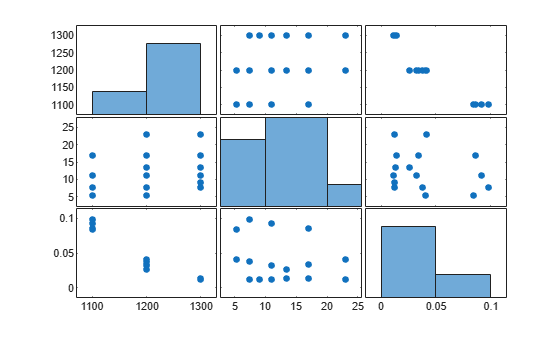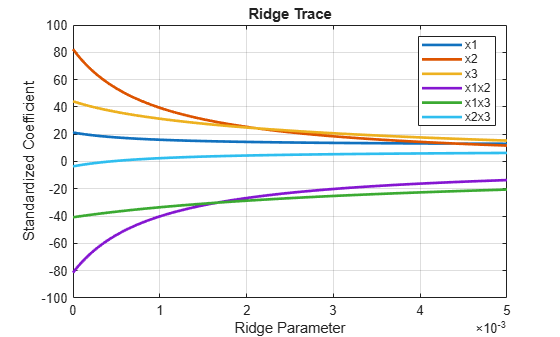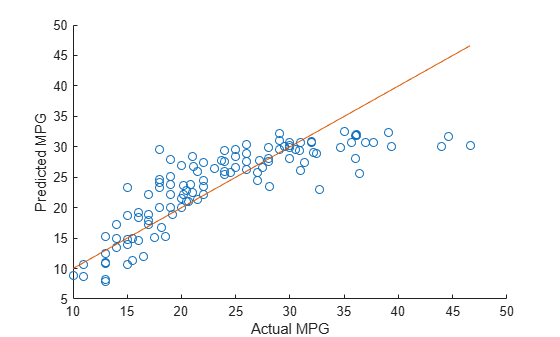Main Content

# ridge

Ridge regression

## Syntax

``B = ridge(y,X,k)``
``B = ridge(y,X,k,scaled)``

## Description

example

````B = ridge(y,X,k)` returns coefficient estimates for ridge regression models of the predictor data `X` and the response `y`. Each column of `B` corresponds to a particular ridge parameter `k`. By default, the function computes `B` after centering and scaling the predictors to have mean 0 and standard deviation 1. Because the model does not include a constant term, do not add a column of 1s to `X`.```

example

````B = ridge(y,X,k,scaled)` specifies the scaling for the coefficient estimates in `B`. When `scaled` is `1` (default), `ridge` does not restore the coefficients to the original data scale. When `scaled` is `0`, `ridge` restores the coefficients to the scale of the original data. For more information, see Coefficient Scaling.```

## Examples

collapse all

Perform ridge regression for a range of ridge parameters and observe how the coefficient estimates change.

Load the `acetylene` data set.

`load acetylene`

`acetylene` contains observations for the predictor variables `x1`, `x2`, and `x3`, and the response variable `y`.

Plot the predictor variables against each other. Observe any correlation between the variables.

`plotmatrix([x1 x2 x3])`For example, note the linear correlation between `x1` and `x3`.

Compute coefficient estimates for a multilinear model with interaction terms, for a range of ridge parameters. Use `x2fx` to create interaction terms and `ridge` to perform ridge regression.

```X = [x1 x2 x3]; D = x2fx(X,'interaction'); D(:,1) = []; % No constant term k = 0:1e-5:5e-3; B = ridge(y,D,k);```

Plot the ridge trace.

```figure plot(k,B,'LineWidth',2) ylim([-100 100]) grid on xlabel('Ridge Parameter') ylabel('Standardized Coefficient') title('Ridge Trace') legend('x1','x2','x3','x1x2','x1x3','x2x3')```The estimates stabilize to the right of the plot. Note that the coefficient of the `x2x3` interaction term changes sign at a value of the ridge parameter $\approx 5*1{0}^{-4}$ .

Predict miles per gallon (MPG) values using ridge regression.

Load the `carbig` data set.

```load carbig X = [Acceleration Weight Displacement Horsepower]; y = MPG;```

Split the data into training and test sets.

```n = length(y); rng('default') % For reproducibility c = cvpartition(n,'HoldOut',0.3); idxTrain = training(c,1); idxTest = ~idxTrain;```

Find the coefficients of a ridge regression model (with k = 5).

```k = 5; b = ridge(y(idxTrain),X(idxTrain,:),k,0);```

Predict `MPG` values for the test data using the model.

`yhat = b(1) + X(idxTest,:)*b(2:end);`

Compare the predicted values to the actual miles per gallon (MPG) values using a reference line.

```scatter(y(idxTest),yhat) hold on plot(y(idxTest),y(idxTest)) xlabel('Actual MPG') ylabel('Predicted MPG') hold off```## Input Arguments

collapse all

Response data, specified as an n-by-1 numeric vector, where n is the number of observations.

Data Types: `single` | `double`

Predictor data, specified as an n-by-p numeric matrix. The rows of `X` correspond to the n observations, and the columns of `X` correspond to the p predictors.

Data Types: `single` | `double`

Ridge parameters, specified as a numeric vector.

Example: `[0.2 0.3 0.4 0.5]`

Data Types: `single` | `double`

Scaling flag that determines whether the coefficient estimates in `B` are restored to the scale of the original data, specified as either `0` or `1`. If `scaled` is `0`, then `ridge` performs this additional transformation. In this case, `B` contains p+1 coefficients for each value of `k`, with the first row of `B` corresponding to a constant term in the model. If `scaled` is `1`, then the software omits the additional transformation, and `B` contains p coefficients without a constant term coefficient.

## Output Arguments

collapse all

Coefficient estimates, returned as a numeric matrix. The rows of `B` correspond to the predictors in `X`, and the columns of `B` correspond to the ridge parameters `k`.

If `scaled` is `1`, then `B` is a p-by-m matrix, where m is the number of elements in `k`. If `scaled` is `0`, then `B` is a (p+1)-by-m matrix.

## More About

collapse all

### Ridge Regression

Ridge regression is a method for estimating coefficients of linear models that include linearly correlated predictors.

Coefficient estimates for multiple linear regression models rely on the independence of the model terms. When terms are correlated and the columns of the design matrix X have an approximate linear dependence, the matrix (XTX)–1 is close to singular. Therefore, the least-squares estimate

`$\stackrel{^}{\beta }={\left({X}^{T}X\right)}^{-1}{X}^{T}y$`

is highly sensitive to random errors in the observed response y, producing a large variance. This situation of multicollinearity can arise, for example, when you collect data without an experimental design.

Ridge regression addresses the problem of multicollinearity by estimating regression coefficients using

`$\stackrel{^}{\beta }={\left({X}^{T}X+kI\right)}^{-1}{X}^{T}y$`

where k is the ridge parameter and I is the identity matrix. Small, positive values of k improve the conditioning of the problem and reduce the variance of the estimates. While biased, the reduced variance of ridge estimates often results in a smaller mean squared error when compared to least-squares estimates.

### Coefficient Scaling

The scaling of the coefficient estimates for the ridge regression models depends on the value of the `scaled` input argument.

Suppose the ridge parameter k is equal to 0. The coefficients returned by `ridge`, when `scaled` is equal to `1`, are estimates of the bi1 in the multilinear model

yμy = b11z1 + ... + bp1zp + ε

where zi = (xiμi)/σi are the centered and scaled predictors, yμy is the centered response, and ε is an error term. You can rewrite the model as

y = b00 + b10x1 + ... + bp0xp + ε

with ${b}_{0}^{0}={\mu }_{y}-\sum _{i=1}^{p}\frac{{b}_{i}^{1}{\mu }_{i}}{{\sigma }_{i}}$ and ${b}_{i}^{0}=\frac{{b}_{i}^{1}}{{\sigma }_{i}}$. The bi0 terms correspond to the coefficients returned by `ridge` when `scaled` is equal to `0`.

More generally, for any value of `k`, if ```B1 = ridge(y,X,k,1)```, then

``` m = mean(X); s = std(X,0,1)'; B1_scaled = B1./s; B0 = [mean(y)-m*B1_scaled; B1_scaled]```

where `B0 = ridge(y,X,k,0)`.

## Tips

• `ridge` treats `NaN` values in `X` or `y` as missing values. `ridge` omits observations with missing values from the ridge regression fit.

• In general, set `scaled` equal to `1` to produce plots where the coefficients are displayed on the same scale. See Ridge Regression for an example using a ridge trace plot, where the regression coefficients are displayed as a function of the ridge parameter. When making predictions, set `scaled` equal to `0`. For an example, see Predict Values Using Ridge Regression.

## Alternative Functionality

• Ridge, lasso, and elastic net regularization are all methods for estimating the coefficients of a linear model while penalizing large coefficients. The type of penalty depends on the method (see More About for more details). To perform lasso or elastic net regularization, use `lasso` instead.

• If you have high-dimensional full or sparse predictor data, you can use `fitrlinear` instead of `ridge`. When using `fitrlinear`, specify the `'Regularization','ridge'` name-value pair argument. Set the value of the `'Lambda'` name-value pair argument to a vector of the ridge parameters of your choice. `fitrlinear` returns a trained linear model `Mdl`. You can access the coefficient estimates stored in the `Beta` property of the model by using `Mdl.Beta`.

 Hoerl, A. E., and R. W. Kennard. “Ridge Regression: Biased Estimation for Nonorthogonal Problems.” Technometrics. Vol. 12, No. 1, 1970, pp. 55–67.

 Hoerl, A. E., and R. W. Kennard. “Ridge Regression: Applications to Nonorthogonal Problems.” Technometrics. Vol. 12, No. 1, 1970, pp. 69–82.

 Marquardt, D. W. “Generalized Inverses, Ridge Regression, Biased Linear Estimation, and Nonlinear Estimation.” Technometrics. Vol. 12, No. 3, 1970, pp. 591–612.

 Marquardt, D. W., and R. D. Snee. “Ridge Regression in Practice.” The American Statistician. Vol. 29, No. 1, 1975, pp. 3–20.

Download ebook